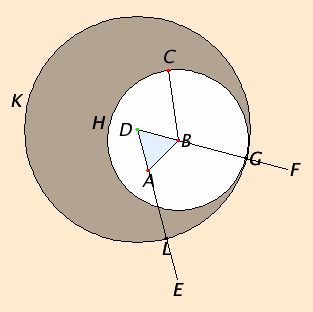# Proposition 2

To place a straight line equal to a given straight line with one end at a given point.

Let A be the given point, and BC the given straight line.It is required to place a straight line equal to the given straight line BC with one end at the point A.

Join the straight line AB from the point A to the point B, and construct the equilateral triangle DAB on it.

Produce the straight lines AE and BF in a straight line with DA and DB. Describe the circle CGH with center B and radius BC, and again, describe the circle GKL with center D and radius DG.

I.Def.15

Since the point B is the center of the circle CGH, therefore BC equals BG. Again, since the point D is the center of the circle GKL, therefore DL equals DG.

C.N.3

And in these DA equals DB, therefore the remainder AL equals the remainder BG.

C.N.1

But BC was also proved equal to BG, therefore each of the straight lines AL and BC equals BG. And things which equal the same thing also equal one another, therefore AL also equals BC.

Therefore the straight line AL equal to the given straight line BC has been placed with one end at the given point A.

Q.E.F.

## Guide

This is a very clever construction to solve what seems to be a simple problem. One would like simply to slide the line BC along so that one end coincides with the point A. But there is no motion in the geometry of Euclid. There is something like motion used in proposition I.4, but nothing is actually moved there. The only basic constructions that Euclid allows are those described in Postulates 1, 2, and 3. Euclid then builds new constructions (such as the one in this proposition) out of previously described constructions. So at this point, the only constructions available are those of the three postulates and the construction in proposition I.1, and Euclid uses all four here.

Another, different, expectation is that one might use a compass to transfer the distance BC over to the point A. It is clear from Euclid’s use of postulate 3 that the point to be used for the center and a point that will be on the circumference must be constructed before applying the postulate; postulate 3 is not used to transfer distance. Sometimes postulate 3 is likened to a collapsing compass, that is, when the compass is lifted off the drawing surface, it collapses.

It could well be that in some earlier Greek geometric theory abstracted compasses that could transfer distances. If that speculation is correct, then this proposition would be a late addition to the theory. The construction of the proposition allows a weaker postulate (namely postulate 3) to be assumed.

#### Construction steps

When using a compass and a straightedge to perform this construction there are more circles drawn than shown in the diagram that accompanies the proposition. These are the two circles needed to construct the equilateral triangle ABD. One side, AB, of that triangle isn’t necessary for the construction.

Altogether, four circles and two lines are required for this construction.#### Use of Proposition 2

The construction in this proposition is only used in Proposition I.3.

Note that this construction assumes that all the point A and the line BC lie in a plane. It may also be used in space, however, since Proposition XI.2 implies that A and BC do lie in a plane.